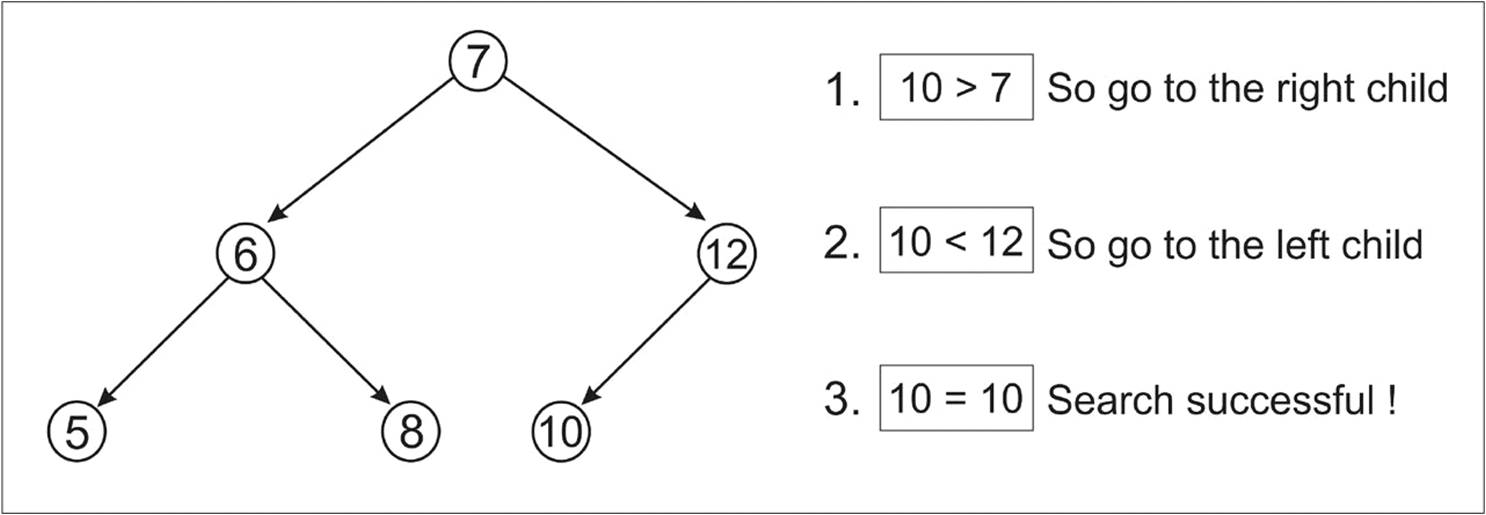# Searching a node in a Binary Search Tree

## Searching a data value in a Binary Search Tree. It says whether the data value is present or not in a Tree.

Searching a data value in BST is similar to search function.

## Algorithm

If an element to be searched
1. If root is Null then return false. Otherwise,
2. If root value is equal to the searched value then return True
3. If searched value is less than or equal to the root’s value, recursively search in left subtree, Else
4. If searched value is greater than the root’s value, recursively search in right subtree.

Time Complexity

The worst case time complexity of a Search operation O(h) is h. where h is height of Binary Search Tree

### Example

figure : Searching value 10 in given Tree## C++ Program

( which cannot be compiled )

```bool Search(Node* root, int data)
{
if(root = NULL){		//If root is Null return false, as there is no element in tree
return false;
}
else if(data = root->data){ // If root data is equal to the searched data, then return true
return true;
}
else if(data <= root->data){ //If the searched data is less than or equal to root data, recursively search in left tree
return Search(root->left,data);
}
else{
return Search(root->right,data); // If the searched data is greater than root data, recursively search in right tree
}
}```

## final code for both search and insert

```#include<iostream>
using namespace std;
//Definition of Node for Binary search tree
struct Node {
int data;
Node* left;
Node* right;
};

Node* NewNode(int data) {   // Function to create a new Node
Node* newNode = new Node();
newNode->data = data;
newNode->left = newNode->right = NULL;
return newNode;
}

Node* Insert(Node* root,int data) {  // To insert data values in BST, This function returns address of root node
if(root == NULL) { 	// empty tree
root = NewNode(data);
}
else if(data <= root->data) {   // if data to be inserted is lesser, insert in left subtree in a recursive manner.
root->left = Insert(root->left,data);
}
else {							// else, insert in right subtree in a recursive manner.
root->right = Insert(root->right,data);
}
return root;
}

bool Search(Node* root,int data) {  //return true if the data is present, else false.
if(root == NULL) {              //If root is Null return false, as there is no element in tree
return false;
}
else if(root->data == data) {  // If root data is equal to the searched data, then return true
return true;
}
else if(data <= root->data) {   //If the searched data is less than or equal to root data, recursively search in left tree
return Search(root->left,data);
}
else {							// If the searched data is greater than root data, recursively search in right tree
return Search(root->right,data);
}
}
int main() {
Node* root = NULL;  // Creating an empty tree
/* Forming a Tree with values 20,40,30,60,10 */
root = Insert(root,20);
root = Insert(root,40);
root = Insert(root,30);
root = Insert(root,60);
root = Insert(root,10);

if(Search(root,40) == true) cout<<"Found\n";  //example....searching whether 40 is present or not